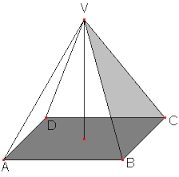Calculate the volume of a regular quadrilateral pyramid, whose wall height is w = 12 cm and the edge of the base is a = 5 cm.

V =  97.8058 cm3

### Step-by-step explanation:Did you find an error or inaccuracy? Feel free to write us. Thank you!

Tips for related online calculators
The Pythagorean theorem is the base for the right triangle calculator.

#### Grade of the word problem:

We encourage you to watch this tutorial video on this math problem: